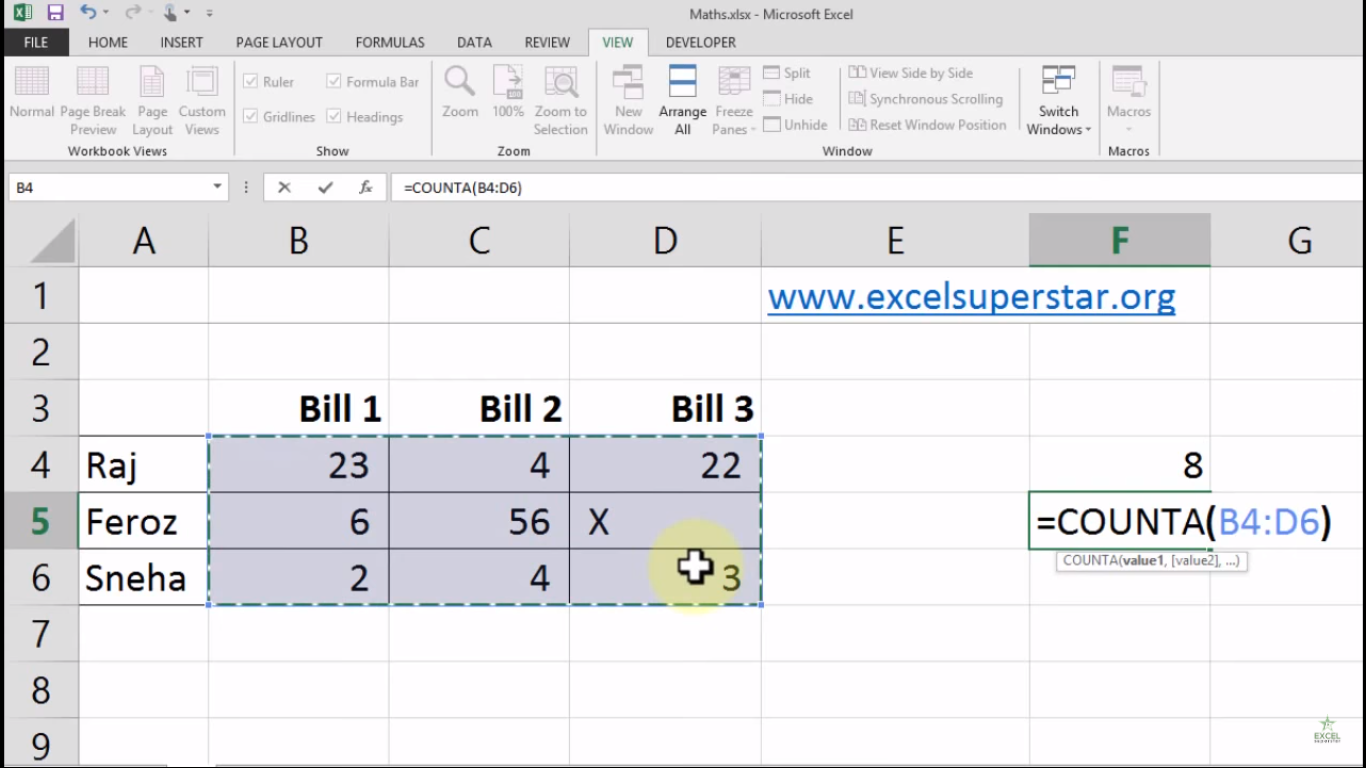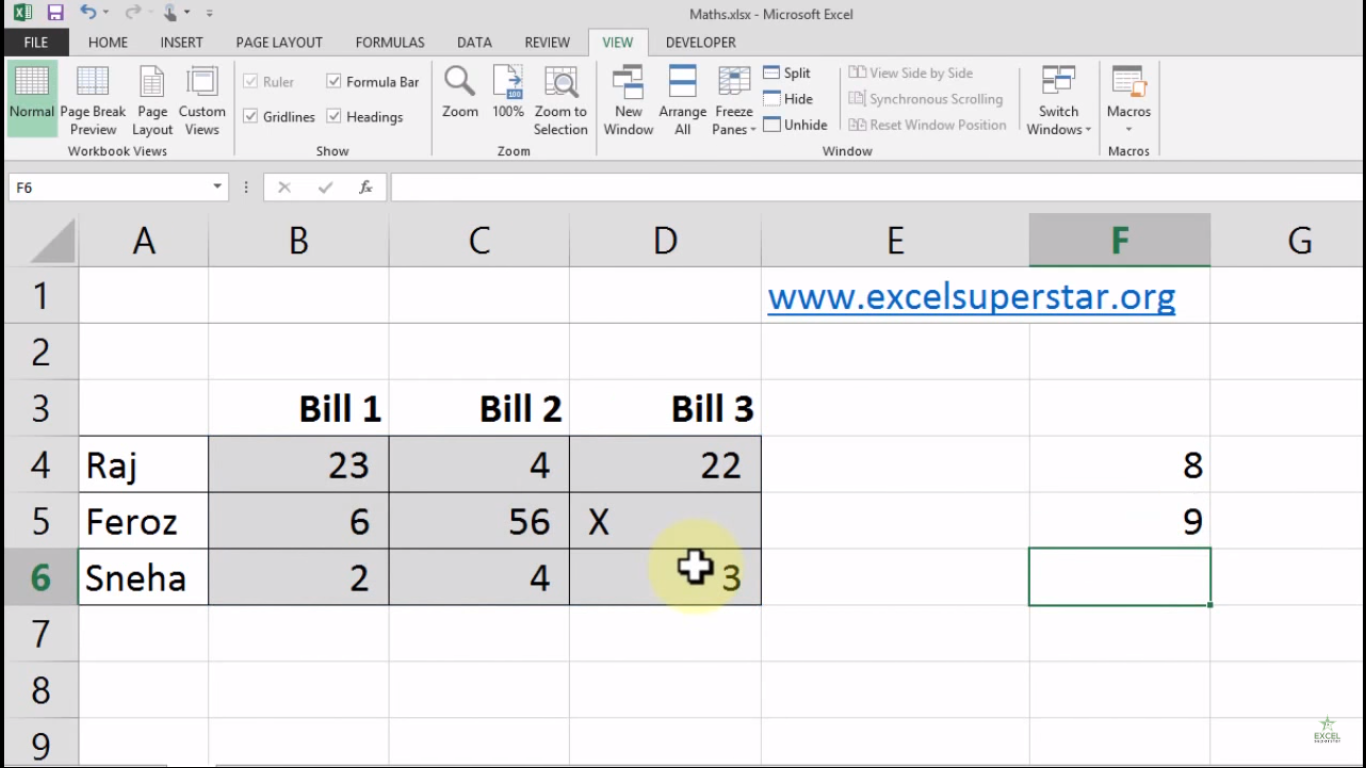# Excel COUNT and COUNTA FormulaIn this tutorial we will learn and apply 2 Formula

1. Count Formula – It counts the number of cells that contain numbers, and counts numbers within the list of arguments.
2. Counta Formula – It counts the number of cells that are not empty in a range

1. Count Formula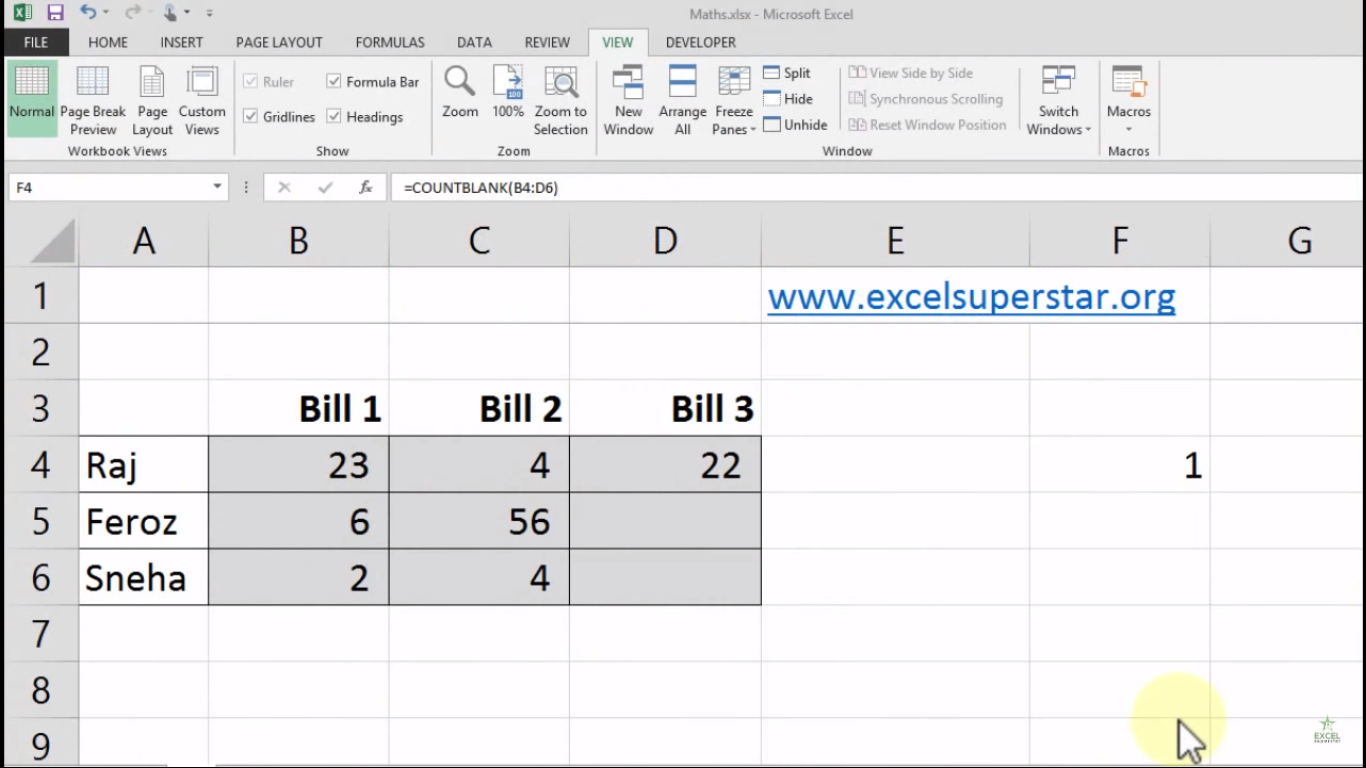There is a table that consists of numbers colored in grey. Now I want to count the number of cells that contains numbers from the given table.

So to calculate the number of cells that contain numbers we will be applying Count Formula:

1. Write =count and press the Tab Key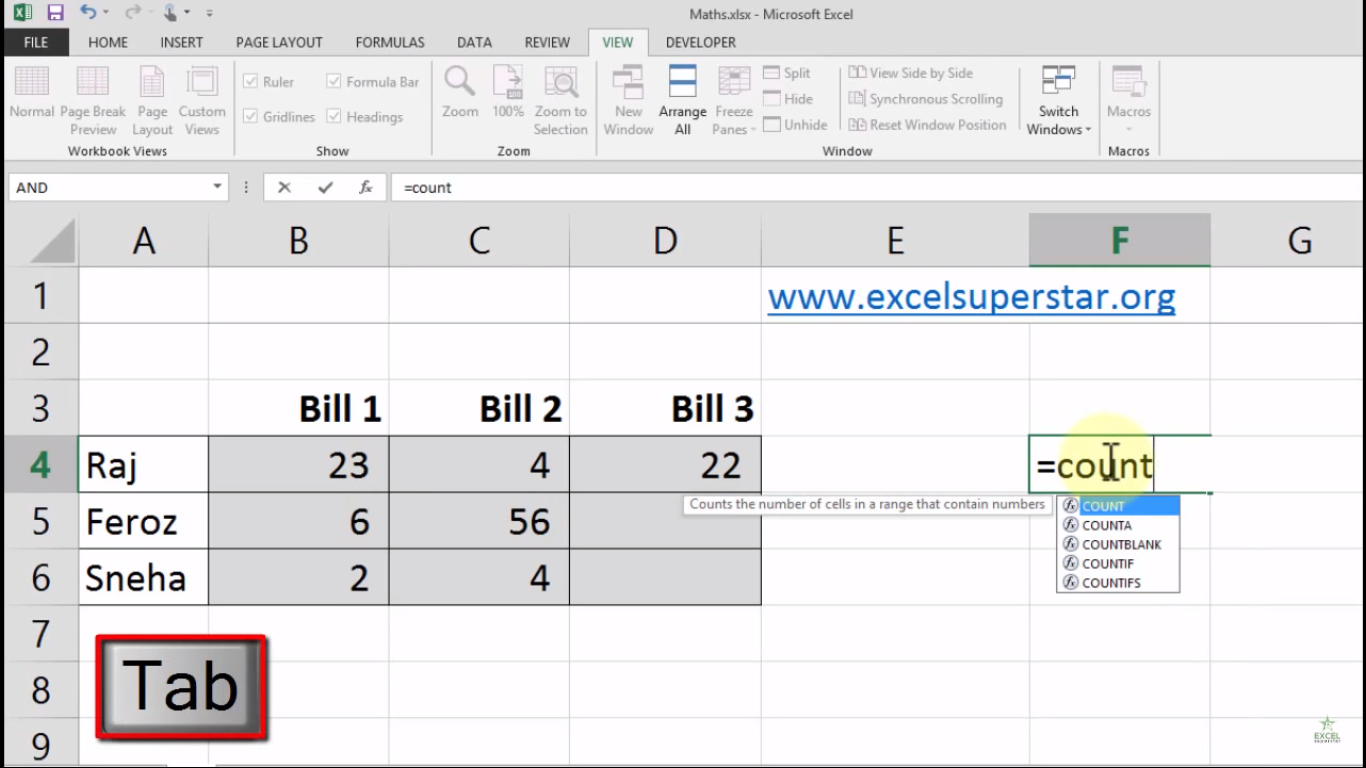2. Select all the grey colored cells from the table and close the bracket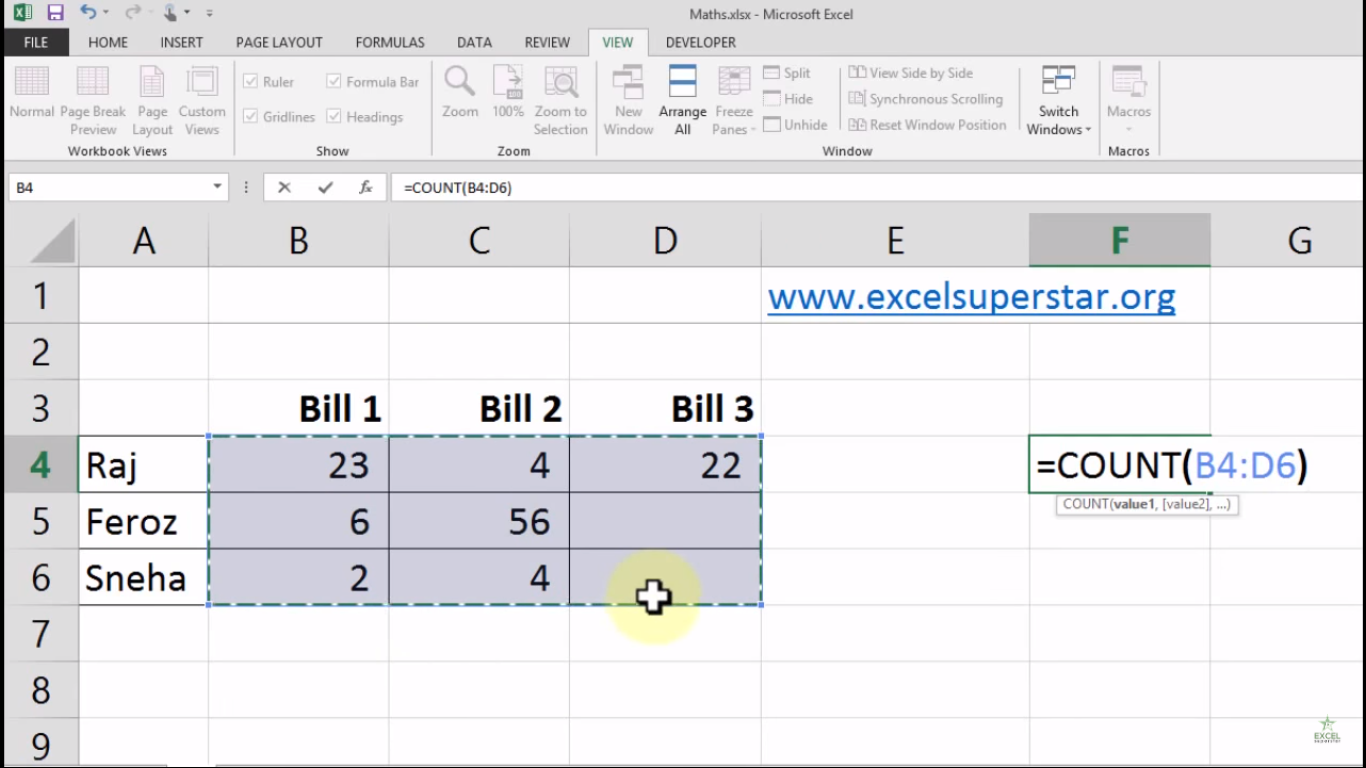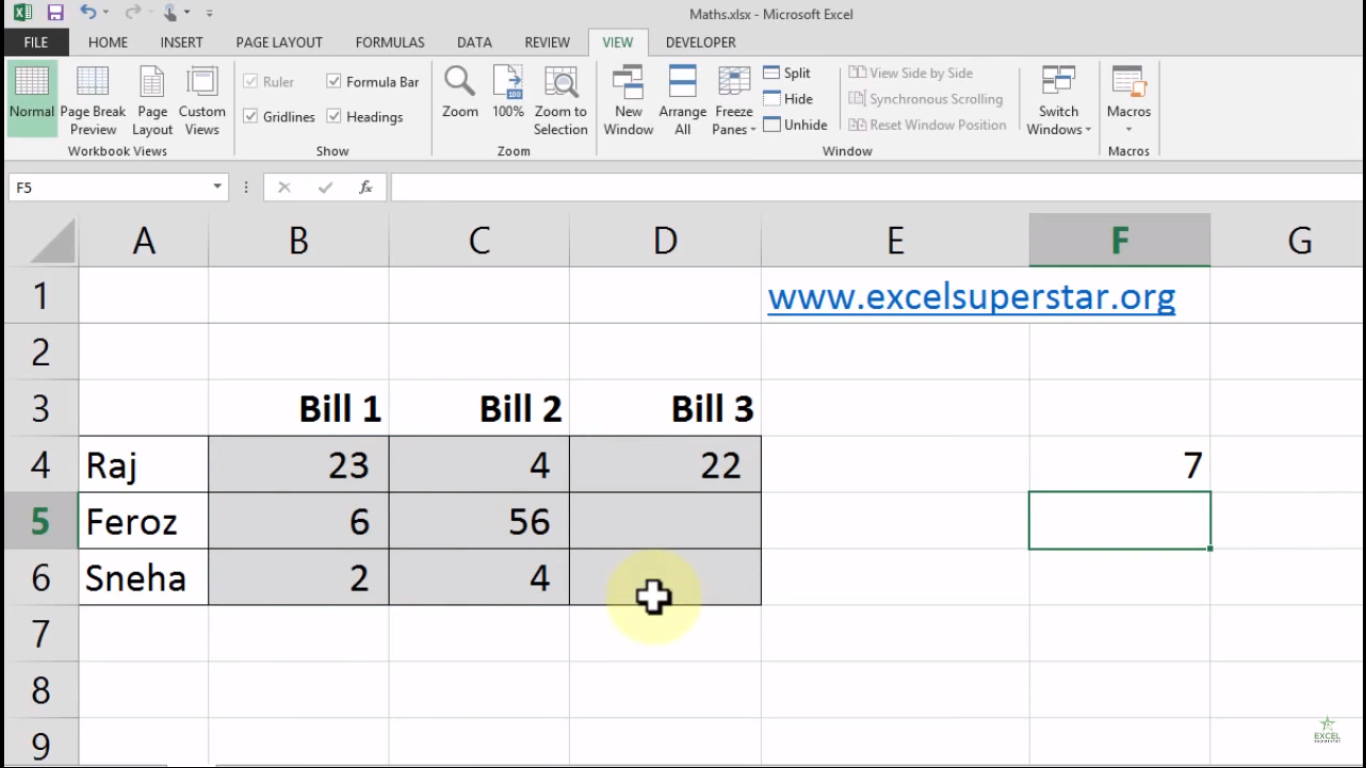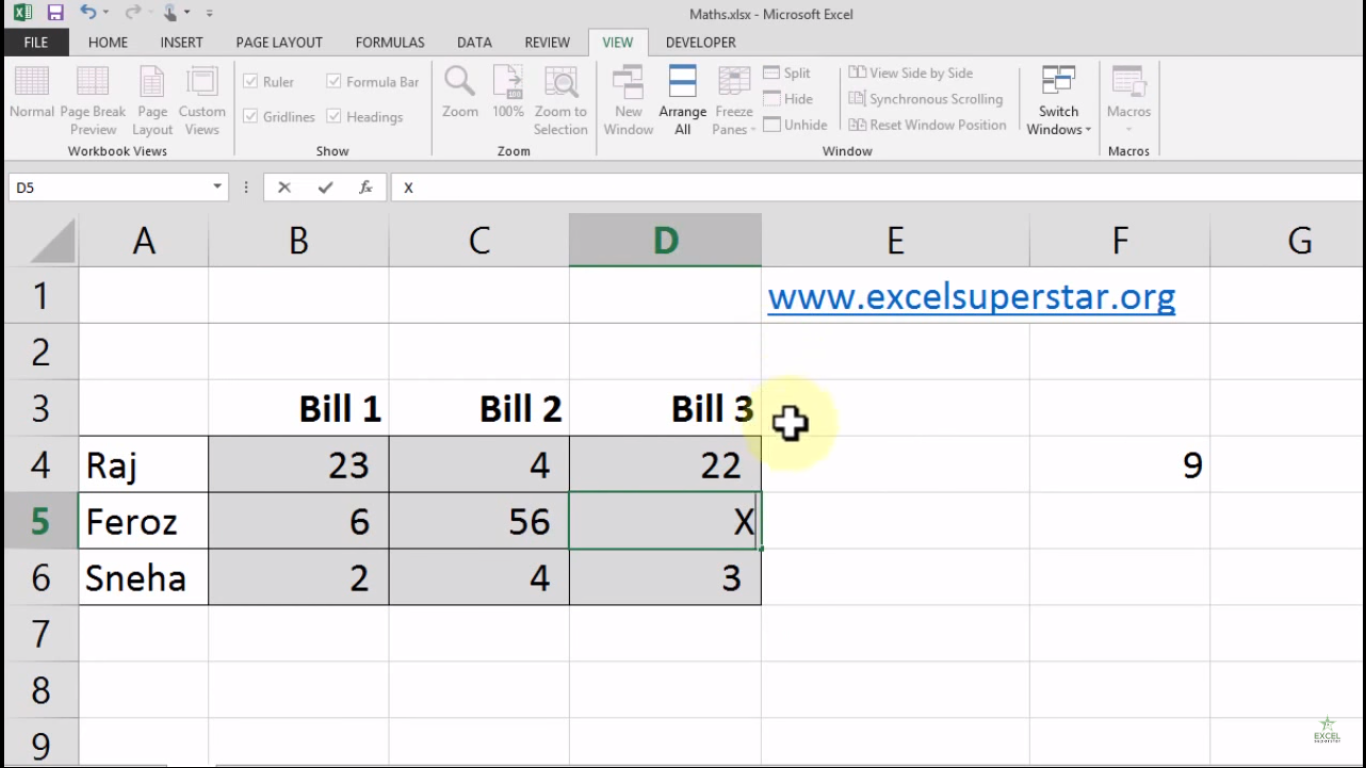2. Counta Formula

Here, if I have a text or character in a given table and want to count the number of cells that are not empty in range then we will be applying Counta Formula:

1. Write =counta and press the Tab Key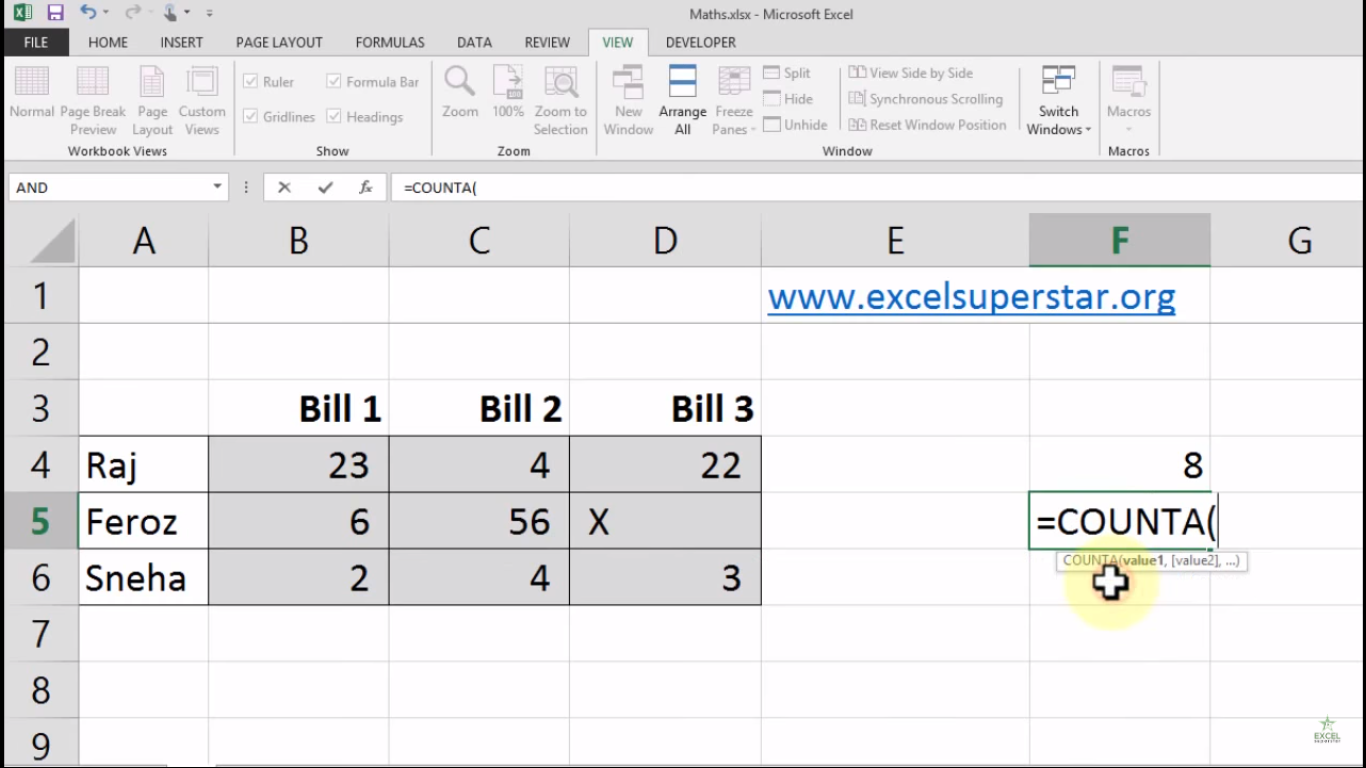2. Select all the grey colored cells from the table and close the bracket